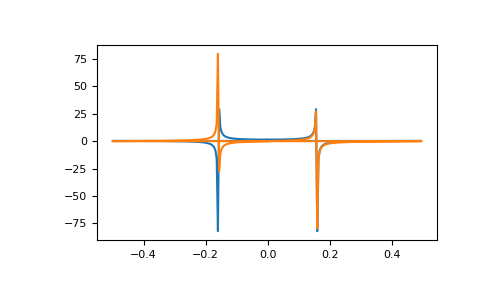# numpy.fft.fft¶

`numpy.fft.``fft`(a, n=None, axis=-1, norm=None)[source]

Compute the one-dimensional discrete Fourier Transform.

This function computes the one-dimensional n-point discrete Fourier Transform (DFT) with the efficient Fast Fourier Transform (FFT) algorithm [CT].

Parameters: a : array_like Input array, can be complex. n : int, optional Length of the transformed axis of the output. If n is smaller than the length of the input, the input is cropped. If it is larger, the input is padded with zeros. If n is not given, the length of the input along the axis specified by axis is used. axis : int, optional Axis over which to compute the FFT. If not given, the last axis is used. norm : {None, “ortho”}, optional New in version 1.10.0. Normalization mode (see `numpy.fft`). Default is None. out : complex ndarray The truncated or zero-padded input, transformed along the axis indicated by axis, or the last one if axis is not specified. IndexError if axes is larger than the last axis of a.

`numpy.fft`
for definition of the DFT and conventions used.
`ifft`
The inverse of `fft`.
`fft2`
The two-dimensional FFT.
`fftn`
The n-dimensional FFT.
`rfftn`
The n-dimensional FFT of real input.
`fftfreq`
Frequency bins for given FFT parameters.

Notes

FFT (Fast Fourier Transform) refers to a way the discrete Fourier Transform (DFT) can be calculated efficiently, by using symmetries in the calculated terms. The symmetry is highest when n is a power of 2, and the transform is therefore most efficient for these sizes.

The DFT is defined, with the conventions used in this implementation, in the documentation for the `numpy.fft` module.

References

 [CT] Cooley, James W., and John W. Tukey, 1965, “An algorithm for the machine calculation of complex Fourier series,” Math. Comput. 19: 297-301.

Examples

```>>> np.fft.fft(np.exp(2j * np.pi * np.arange(8) / 8))
array([-2.33486982e-16+1.14423775e-17j,  8.00000000e+00-1.25557246e-15j,
2.33486982e-16+2.33486982e-16j,  0.00000000e+00+1.22464680e-16j,
-1.14423775e-17+2.33486982e-16j,  0.00000000e+00+5.20784380e-16j,
1.14423775e-17+1.14423775e-17j,  0.00000000e+00+1.22464680e-16j])
```

In this example, real input has an FFT which is Hermitian, i.e., symmetric in the real part and anti-symmetric in the imaginary part, as described in the `numpy.fft` documentation:

```>>> import matplotlib.pyplot as plt
>>> t = np.arange(256)
>>> sp = np.fft.fft(np.sin(t))
>>> freq = np.fft.fftfreq(t.shape[-1])
>>> plt.plot(freq, sp.real, freq, sp.imag)
[<matplotlib.lines.Line2D object at 0x...>, <matplotlib.lines.Line2D object at 0x...>]
>>> plt.show()
```#### Previous topic

Discrete Fourier Transform (`numpy.fft`)

numpy.fft.ifft